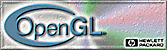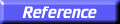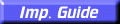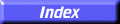# glScale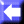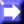glScaled, glScalef: multiply the current matrix by a general scaling matrix.

C Specification | Parameters | Description | Notes | Errors | Associated Gets | See Also

##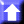C Specification

```void glScaled(
GLdouble	 x,
GLdouble	 y,
GLdouble	 z)
void glScalef(
GLfloat	 x,
GLfloat	 y,
GLfloat	 z)
```

##Parameters

x, y, z
Specify scale factors along the x, y, and z axes, respectively.

##Description

glScale produces a nonuniform scaling along the x, y, and z axes. The three parameters indicate the desired scale factor along each of the three axes.

The current matrix (see glMatrixMode) is multiplied by this scale matrix, and the product replaces the current matrix as if glScale were called with the following matrix as its argument:

 x 0 0 0 0 y 0 0 0 0 z 0 0 0 0 1

If the matrix mode is either GL_MODELVIEW or GL_PROJECTION, all objects drawn after glScale is called are scaled.

Use glPushMatrix and glPopMatrix to save and restore the unscaled coordinate system.

##Notes

If scale factors other than 1 are applied to the modelview matrix and lighting is enabled, lighting often appears wrong. In that case, enable automatic normalization of normals by calling glEnable with the argument GL_NORMALIZE.

##Errors

• GL_INVALID_OPERATION is generated if glScale is executed between the execution of glBegin and the corresponding execution of glEnd.

##Associated Gets

glGet with argument GL_MATRIX_MODE
glGet with argument GL_MODELVIEW_MATRIX
glGet with argument GL_PROJECTION_MATRIX
glGet with argument GL_TEXTURE_MATRIX

glMatrixMode,
glMultMatrix,
glPushMatrix,
glRotate,
glTranslate